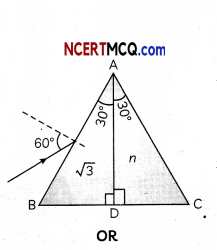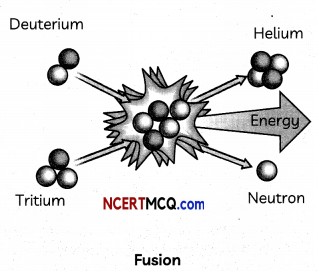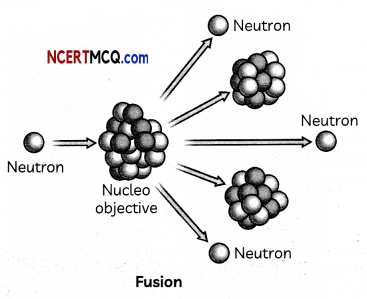Students can access the CBSE Sample Papers for Class 12 Physics with Solutions and marking scheme Term 2 Set 12 will help students in understanding the difficulty level of the exam.

## CBSE Sample Papers for Class 12 Physics Standard Term 2 Set 11 for Practice

Time Allowed: 2 Hours
Maximum Marks: 40

General Instructions:

• There are 12 questions in all. All questions are compulsory.
• This question paper has three sections: Section A, Section B and Section C.
• Section A contains three questions of two marks each, Section B contains eight questions of three marks each, Section C contains one case study-based question of five marks.
• There is no overall choice. However, an internal choice has been provided in one question of two marks and two questions of three marks. You have to attempt only one of the chokes in such questions.
• You may use log tables if necessary but use of calculator is not allowed.

SECTION – A
(Section A contains 3 questions of 2 marks each.)

Question 1.
What kind of fringes will be observed if we replace monochromatic light by white light?
OR
In a single-slit diffraction experiment, the width of the slit is made double the original width. How does this affect the size and intensity of the central diffraction band? (2)

Question 2.
How does the process of conduction takes place in n-type semiconductors and p-type semiconductors? (2)Question 3.
How would the ionization energy change when electron in hydrogen atom is replaced by a particle of mass 100 times that of the electron but having the same charge? (2)

SECTION – B
(Section B consists of 8 questions of 3 marks each.)

Question 4.
A proton and an alpha particle are accelerated through the same potential? Which one of the two has:
(A) greater value of de-Broglie wavelength associated with it and
(B) less kinetic energy.

Question 5.
A convex lens made up of glass of refractive index 1.5 is dipped, in turn, in:
(A) a medium of refractive index 1.65,
(B) a medium of refractive index 1.33.
(i) Will it behave as a converging or a diverging lens in the two cases?
(ii) How will its focal length change in the two media? (3)

Question 6.
(A) Draw a labelled ray diagram showing the formation of a final image by a compound microscope at least distance of distinct vision.
(B) The total magnification produced by a compound microscope is 20. The magnification produced by the eye piece is 5. The microscope is focused on a certain object. The distance between the objective and eye-piece is observed to be 14 cm. If least distance of distinct vision is 20 cm, calculate the focal length of the objective and the eye-piece. (3)Question 7.
A 12.3 eV electron beam is used to bombard gaseous hydrogen at room temperature. Up to which energy level the hydrogen atoms would be excited? Calculate the wavelength of the second member of Lyman series and second member of Balmer series. (3)

Question 8.
Induced electric field due to changing magnetic flux are more readily observed than induced magnetic field due to changing electric field. Why?
OR
State the laws of photoelectric emission. (3)

Question 9.
Why are Si and GaAs are preferred for making solar cells? Briefly explain working principle of a solar cell with the help of a necessary circuit diagram. (3)Question 10.
What is a rectifier? Draw a labelled diagram
of Full-wave rectifier and its input and out¬put waveform. (3)

Question 11.
A composite prism ABC is made of two identical right-angled prisms ABD and ADC made of different materials of refractive indices √3 andm respectively. A ray of light is incident on face AB of the prism at 60° and the emergent ray grazes along the face AC. Find the value of n.Consider two coherent sources S1 and S2 producing monochromatic waves to produce interference pattern. Let the displacement of the wave produced by S1 be given by Y1 = a cos ωt and the displacement by S2 be Y2 = a cos (ωt + Φ). Find out the expression for the amplitude of the resultant displacement at a point and show that the intensity at
that point will be I = 4a2 cos2 $$\frac{\phi}{2}$$. Hence
establish the conditions for constructive and destructive interference. (3)SECTION – C
(Section C consists one case study-based question of 5 marks.)

Question 12.
There wilt be a gain in the overall binding energy when we move from the heavy nuclei region to the middle region of the plot, hence release of energy when a heavy nucleus (A = 240) breaks into two roughly equal fragments, energy can be released. This process is called nuclear fission. Similarly, there will be gain in(A) The binding energy per nucleon for the parent nucleus is E1 and that for the daughter nuclei is E2. Then A nucleus of mass M + Am is at rest and decays into two daughter nuclei of equal mass M/2 each. Speed of light is c.
(i) E1 = 2E2
(ii) E2 = 2E1
(iii) E1 > E2
(iv) E2 > E1
the overall binding energy, when we move from lighter nuclei to heavier nuclei, hence release of energy. When two or more lighter nuclei fuse together to form a heavy nucleus energy can be released. This process is called Nuclear Fusion. This is the energy source of sun.(B) Solar energy is mainly caused due to:
(i) burning of hydrogen in the oxygen
(ii) fission of uranium present in the sun
(iii) fusion of protons during synthesis of heavier elements
(iv) gravitational contraction(C) 200 MeV of energy may be obtained per fission of 235U. A reactor is generating
1000 KW of power. The rate of nuclear fission in the reactor is:
(i) 1000
(ii) 2 × 108
(iii) 3.125 × 1016
(iv) 931
(D) Which type of reaction produces the most harmful radiation?
(i) Fusion reaction
(ii) Chemical reaction
(iii) Fission reaction
(iv) Photo-Chemical reaction

(E) The average energy released per fission of $$\begin{gathered} 235 \\ 92 \end{gathered}$$U is: ,
(i) 200 eV 00
(ii) 200 MeV
(iii) 400 meV
(Vi) 200 GeV (5)